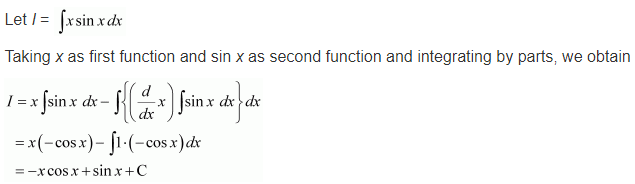# NCERT Solutions for Class 12 Maths Chapter 7 Integers Ex 7.6

Get Free NCERT Solutions for Class 12 Maths Chapter 7 Integers Ex 7.6 PDF. Integration Class 12 Maths NCERT Solutions are extremely helpful while doing your homework. Exercise 7.6 Class 12 Maths NCERT Solutions were prepared by Experienced LearnCBSE.in Teachers. Detailed answers of all the questions in Chapter 7 Maths Class 12 Integrals Exercise 7.6 provided in NCERT Textbook.

The topics and sub-topics included in the Integrals chapter are the following:

 Section Name Topic Name 7 Integrals 7.1 Introduction 7.2 Integration as an Inverse Process of Differentiation 7.3 Methods of Integration 7.4 Integrals of some Particular Functions 7.5 Integration by Partial Fractions 7.6 Integration by Parts 7.7 Definite Integral 7.8 Fundamental Theorem of Calculus 7.9 Evaluation of Definite Integrals by Substitution 7.10 Some Properties of Definite Integrals

## NCERT Solutions for Class 12 Maths Chapter 7 Integers Ex 7.6

NCERT Solutions for Class 12 Maths Chapter 7 Integers Ex 7.6 are part of NCERT Solutions for Class 12 Maths. Here we have given Class 12 Maths NCERT Solutions Integrals Ex 7.6

Integrate the functions in Exercises 1 to 22.
Question 1.
x sinx
Solution:Question 2.
x sin3x
Solution:Question 3.${ x }^{ 2 }{ e }^{ x }$
Solution:Question 4.
x logx
Solution:Question 5.
x log2x
Solution:Question 6.${ x }^{ 2 }logx$
Solution:Question 7.$x\quad { sin }^{ -1 }x$
Solution:Question 8.$x\quad { tan }^{ -1 }x$
Solution:Question 9.$x\quad { cos }^{ -1 }x$
Solution:Question 10.${ (sin }^{ -1 }{ x })^{ 2 }$
Solution:Question 11.$\frac { x\quad { cos }^{ -1 }x }{ \sqrt { 1-{ x }^{ 2 } } }$
Solution:Question 12.
x sec²x
Solution:Question 13.${ ta }n^{ -1 }x$
Solution:Question 14.
x(logx)²
Solution:Question 15.
(x²+1)logx
Solution:Question 16.${ e }^{ x }(sinx+cosx)$
Solution:Question 17.$\frac { { xe }^{ x } }{ { (1+x) }^{ 2 } }$
Solution:Question 18.$\frac { { e }^{ x }(1+sinx) }{ 1+cosx }$
Solution:Question 19.${ e }^{ x }\left( \frac { 1 }{ x } -\frac { 1 }{ { x }^{ 2 } } \right)$
Solution:Question 20.$\frac { { (x-2)e }^{ x } }{ { (x-1) }^{ 3 } }$
Solution:Question 21.${ e }^{ 2x }sinx$
Solution:Question 22.${ sin }^{ -1 }\left( \frac { 2x }{ 1+{ x }^{ 2 } } \right)$
Solution:Choose the correct answer in exercise 23 and 24

Question 23.$\int { { x }^{ 2 }{ e }^{ { x }^{ 3 } } } dx\quad equals$
(a)$\frac { 1 }{ 3 } { e }^{ { x }^{ 3 } }+c$
(b)$\frac { 1 }{ 3 } +{ e }^{ { x }^{ 2 } }+c$
(c)$\frac { 1 }{ 2 } { e }^{ { x }^{ 3 } }+c$
(d)$\frac { 1 }{ 2 } { e }^{ { x }^{ 2 } }+c$
Solution:Question 24.$\int { { e }^{ x }secx(1+tanx) } dx\quad equals$
(a)${ e }^{ x }cosx+c$
(b)${ e }^{ x }secx+c$
(c)${ e }^{ x }sinx+c$
(d)${ e }^{ x }tanx+c$
Solution:+